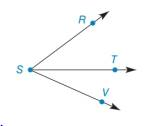Chapter 1.2, Problem 20EElementary Geometry For College St...

7th Edition
Alexander + 2 others
ISBN: 9781337614085

Solutions

Chapter
SectionElementary Geometry For College St...

7th Edition
Alexander + 2 others
ISBN: 9781337614085
Textbook ProblemExercises 16 − 24 Given: m ∠ R S T = 5 ( x + 1 ) − 3 m ∠ T S V = 4 ( x − 2 ) + 3 m ∠ R S V = 4 ( 2 x + 3 ) − 7 Find: x     and m ∠ R S V

To determine

To find:

The value of x and the measure of the angle RSV.

Explanation

Given:

mRST=5(x+1)3mTSV=4(x2)+3mRSV=4(2x+3)7

The given figure is,

Definition:

Angle addition postulate says that,

If a point say M lies in the interior of PQR, then mPQM+mRQM=mPQR.

Calculation:

Consider the figure,

T is the interior point of the angle RSV.

mRST=5(x+1)3mTSV=4(x2)+3mRSV=4(2x+3)7

Use above mentioned angle addition postulate to solve for the value of x.

Still sussing out bartleby?

Check out a sample textbook solution.

See a sample solution

The Solution to Your Study Problems

Bartleby provides explanations to thousands of textbook problems written by our experts, many with advanced degrees!

Get Started

Find more solutions based on key concepts